1. Overview of Thermoelectric Modules

Thermoelectric modules, also known as thermoelectric devices or thermoelectric coolers/generators, are electronic components used in the fields of thermoelectric cooling (TEC) and thermoelectric power generation (TEG). In particular, thermoelectric cooling is widely used in precision temperature control applications where the cooling target needs to be actively cooled to below the ambient temperature or requires temperature control of 0.01℃ or less.

Thermoelectric modules can actively control the temperature of a given object by controlling the supply current of the module. They are characterized by being noiseless, vibration-free, without mechanical moving parts, and capable of switching between cooling and heating by changing the direction of the current.

Thermoelectric modules can operate at temperatures up to a maximum of 200℃, which is determined by the reflow temperature of the solder used in the module. In addition, there exists a maximum temperature difference between the hot side and cold side of the thermoelectric module, and in typical applications, a single stage module can achieve a temperature difference of about 50K.

Under operating conditions, if the current exceeds the maximum current value (Imax), the power loss (I2·R) within the thermoelectric module increases, resulting in a rise in temperature. Therefore, thermoelectric modules should be used under conditions where the current does not exceed the maximum current value.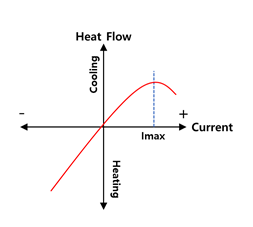Fig 1. Thermal flux varies depending on the current

2. Composition of Thermoelectric Systems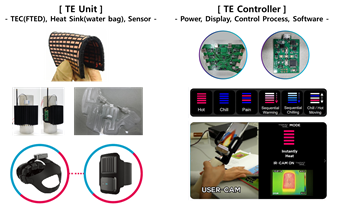Fig 2.  The configuration of a thermoelectric system

A thermoelectric system is composed of thermoelectric modules (also known as thermoelectric coolers/generators), a heat sink (heat plate and/or fan), and a controller. The ambient temperature of the system plays an important role in the performance of the thermoelectric system, which is related to the heat dissipation capacity of the heat sink.

In addition, there are temperature sensors attached to the target object and heat sink, software for monitoring, and a power supply unit.

3. Thermoelectric System's Heat Circuit

Thermoelectric systems typically have a heat circuit connected in series thermally from the cooling target to the heat sink. The diagram below shows a thermal circuit assuming that there is no convective heat loss when the target is perfectly insulated, where Q represents the heat capacity of each component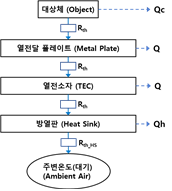Fig 3. Thermoelectric System's Heat Circuit

For example, examining the temperature changes in the heat circuit excluding the heat capacity (Q) of each component, when the cold side of the thermoelectric module is cooled to -5℃, the hot side becomes 35℃ and the heat sink emits heat to the surrounding air at 25℃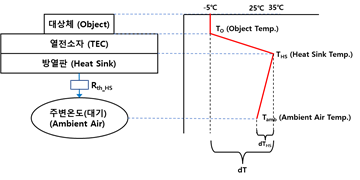Fig 4. The temperature distribution of the thermoelectric system

4. Design a thermoelectric system

To design a thermoelectric system, typically the following steps are taken, although depending on the application, some steps may be repeated, skipped, or modified:

1) Calculate the heat load of the cooling target.

2). Set the operating temperature range for the heat sink and the target.

3) Select thermoelectric modules (TEMs).

4) Select a controller that covers the required power range.

5) Choose a heat sink (optional for heat sink fans).

6) Select temperature sensors.

7) Select a power supply.

In calculating the heat load, the amount of heat (Qc, W) absorbed (or removed) by the cooling (or temperature control) target is important, and there are various types of heat loads depending on the product.

Power consumption Heat transfer rate (or loss): radiation, convection, conduction Dynamic heat load (Dynamic Heat Flow, dQ/dT)

The heat load (Qc) is absorbed on the cold side and transferred to the hot side with the heat sink, and then it is released to the outside (atmosphere).

2) Setting the operating temperature range

When cooling the target object (product) to a given (target) temperature through thermoelectric cooling, the cooled object comes into contact with the cold side of the thermoelectric module and after a certain amount of time, the temperature of the cold side of the thermoelectric module becomes the same as that of the cooled object.

Therefore, the thermoelectric system must set the operating temperature range based on the temperature of the target object and the temperature of the heat sink.

◾ TO: temperature of the target object (cold side temperature, ℃)

◾ THS: temperature of the heat sink (hot side temperature, ℃), THS=Tamb+ΔTHS

◾ dT (ΔT): difference between TO and THS, dT=THS-TO=Tamb+ΔTHS-TO

3) Selection of Thermoelectric Module

○ When selecting a thermoelectric device that generates a temperature difference between both ends of a product due to current flow, the coefficient of performance (COP) is an important criterion to consider.

○ At maximum COP conditions, the thermoelectric device minimizes heat dissipation to the heat sink (Qh=Qc+Pel) with minimum input power. Therefore, the thermoelectric device should be selected to generate the optimal COP under the desired dT conditions.

○ The thermoelectric device should satisfy the maximum heat absorption capacity (Qmax, W) while considering sufficient design margin to suppress thermal runaway caused by changes in ambient temperature or active thermal loads.

○ Key considerations when selecting a thermoelectric device:

◾ Choose a thermoelectric device that is larger than the required heat pump capacity.

◾ Design the system with operating current conditions much lower than the maximum current (Imax) of the thermoelectric device.

◾ Increase the heat dissipation capacity of the heat sink by increasing the size of the heat sink or adding a heat sink fan.

4) Selection of the controller

The controller adjusts the current supplied to the thermoelectric cooler to control the temperature of the cooling target. In this case, the controller is selected based on the supplied current (operating current) of the thermoelectric cooler, not Imax.

5) Selection of Heat Sink

○ The heat sink absorbs heat from the hot side of the thermoelectric module and releases it to the surrounding air, thus determining the operating temperature range and efficiency of the thermoelectric system. Therefore, the heat sink size should be selected with some margin to suppress the temperature rise of the heat sink.

○ As shown in the figure below, the amount of heat generated by the thermoelectric module (Qh, W) can rise up to 2.6 times the maximum heat absorption (Qmax) due to additional heat generated inside the thermoelectric module during the heat pumping process. Therefore, the heat sink should be selected based on the total heat generation, which considers both the heat load of the target object and the additional heat generated inside the thermoelectric module.

○ It is advantageous to estimate the total heat dissipation in the heat sink using the performance curve provided by the thermoelectric module manufacturer. Typically, thermoelectric module manufacturers provide data on heat generation according to current and temperature conditions (temperature difference, dT), and the following figure explains the case of the TEGway S169A068085 model.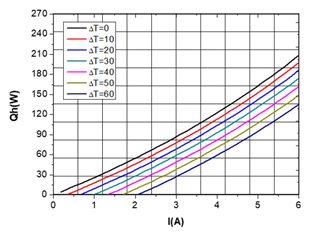Fig 5. The relationship between current and (Qh/Qmax).

(TEGWAY- S169A068085 model)

○ To enhance the cooling performance of a thermoelectric module, the thermal resistance of the heat sink should be low. The thermal resistance of the heat sink is calculated by dividing the temperature difference between the ambient air and the heat sink by the amount of heat flowing into the heat sink, and ΔTHS is calculated based on the maximum ambient temperature.

◾ Rth_HS (K/W) = ΔTHS / Qh

◾ ΔTHS: temperature difference between the heat sink and ambient air (K)

◾ Qh: total heat load (W)

* Note) Relationship between dT and Heat Dissipation (dT-Heat dissipation relationship)

• As the supply current of the thermoelectric module increases, the heat dissipation (Qh) of the thermoelectric module increases, and especially as the dT of the thermoelectric module increases, the amount of heat emitted to the heat sink becomes larger than the amount of heat absorbed from the cold side of the thermoelectric module.

• Therefore, based on the heat transfer rate (Qc) and heat dissipation (Qh) data provided by the thermoelectric module manufacturer according to the input power (current), the actual value according to the temperature difference (dTHS) is predicted and the heat resistance of the heat sink is calculated.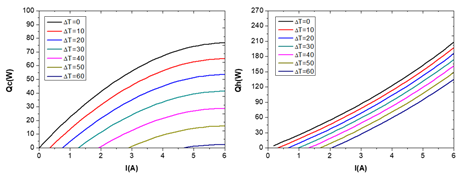Fig 6. Variation of Qc and Qh according to the current

(TEGWAY- S169A068085 model)

◦ Review stage

• Find the values of Qh and Qc according to the given current and dT conditions on the graph.

• Choose the target temperature difference (dTHS) between the heat sink and ambient air temperature.

• Use the relationship equation between Rth_HS and Qh to determine Rth_HS for a given Qh (or Qc): Rth_HS = ΔTHS / (ratio x QC), ratio = Qh/Qc

• The temperature difference (dT) caused by the heat sink's thermal resistance is calculated as follows: dT = Tamb + ΔTHS – TO, where ΔTHS = Qh / Rth_HS.

* Note) Review of Heat sink fan

•  In order to reduce the thermal resistance of the heat sink (from the heat sink to the ambient air), it is necessary to use a heat sink fan, which can improve the performance of the heat sink and reduce its temperature difference (dT) and size.

•  Fan speed control is generally achieved using PWM control, and it is convenient to use the same voltage as the TEC controller.

6) Example of calculating the capacity of thermoelectric module and heat sink

•  In selecting a thermoelectric module for a thermoelectric system design, the two important selection criteria are the maximum cooling capacity (Qmax) and the temperature difference (dT) of the thermoelectric module.

* Reference) Definition of temperature and prediction of thermal load

•  When a target with a thermal load of QC=10W is cooled to 0℃ (TO=0℃) and the ambient temperature is 25℃, assuming that the temperature of the heat sink (TS) is 30℃, the temperature difference between the two ends of the thermoelectric module is 30K.

•  In other words, the temperature difference between the two ends of the thermoelectric module is based on the temperature of the heat sink, not the ambient temperature.

* Reference) Selection of thermoelectric module

•  The thermoelectric module should have a capacity (Qmax) that can achieve the best COP while having the required Qc.

•  In the performance coefficient versus current graph of the thermoelectric module, the condition that has the maximum value of dT=30K at the relative current of 0.45 (I=2.7A) is found. At this time, the current condition is generally searched for at a condition lower than 0.7.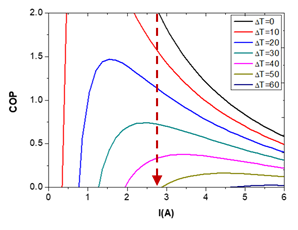Fig 7. The relationship between current and COP

(TEGWAY- S169A068085 model)

•  Using the current condition, the QC value is determined to be 22W with a dT of 30K and a relative current (I/Imax) of 0.45 on the graph below. (Qmax=76W, QC/Qmax=0.28)Fig 8. The relationship between current and heat transfer capacity (Qc)

(TEGWAY- S169A068085 model)

• Using the performance versus current graph, the COP at a relative current (I/Imax) of 0.7 is determined and the input power is calculated: Pel = QC/COP = 22W/0.7 = 31.4W

• Based on the data provided by the thermoelectric module manufacturer, a product that satisfies Qc under the condition of Qmax being greater than 76W and dT being 30K (achievable with a single-stage module) is selected.

• The operating current and voltage are calculated as follows:

• - Operating current, I = Imax*(I/Imax) = 6A*0.45 = 2.7A
• - Operating voltage, V = Pel/I = 31.4W/2.7A = 11.6V

• The controller is selected based on the calculated operating current and voltage, taking into account design margins.

* Reference) Heat Sink

•  To select a suitable heat sink for the thermoelectric module, the thermal resistance of the heat sink should be calculated.

•  The heat dissipation vs. current graph is used to determine Qh under the condition of dT=30K: Qh = 50W (Qh/Qmax = 0.65, Qmax = 76W)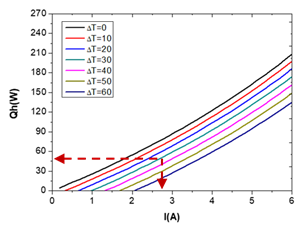Fig 9. The relationship between current and heat dissipation (Qh)

(TEGWAY- S169A068085 model)

•  Calculate the thermal resistance of the heat sink: Rth_HS = ΔTHS/Qh = 5K/50W = 0.1K/W

•  Select a heat sink with a thermal resistance lower than the calculated value of 0.1K/W.

•  To actually design the optimal thermal management system, the design parameters should be determined through iteration and experimentation in the design phase.

7) Others: Temperature Sensors and Power Supplies

•  Temperature sensors are used to measure the temperature of the target object and the heat sink, and various types of temperature sensors, including NTC, TC-K, PT100, and PT1000, are used. To accurately control the temperature of the target object, the temperature sensor is installed as close as possible to the target object to ensure intimate contact.

•  PT100 sensors are commonly used considering their high precision and wide operating temperature range, especially in the case of measuring extremely low temperatures below 0°C, where the increase in resistance value is significant and NTC cannot be used. Note that the resistance value of the sensor should be selected to be smaller than the reference resistance of the controller.

•  The power supply should be selected with a capacity that has about 10% margin for the required TEC output power. At this time, the power supply should also consider the power required for driving the controller along with TEC output.